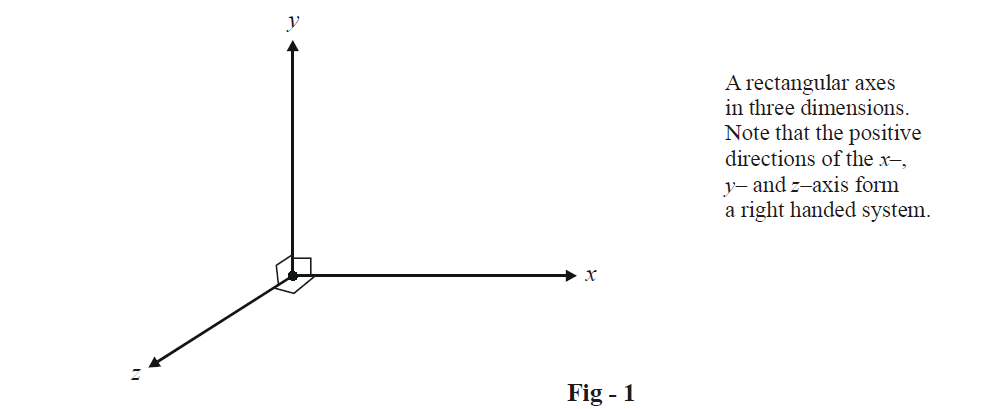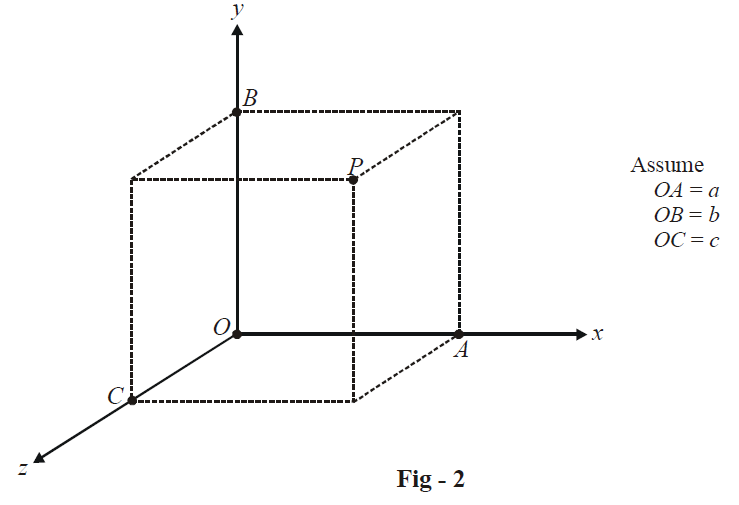# Three Dimensional Coordinate Axes

Go back to  'Three Dimensional Geometry'

In our study of coordinate geometry we used coordinates to study planar geometry. We can easily extend the use of coordinates to study geometry in three dimensions; this is what we’ll be doing in this chapter.

Most of the discussion of this chapter follows in a very straight forward manner from our discussions on Vectors; that is the reason for this topic being discussed in the Appendix. It is being assumed in the subsequent discussions that you have studied Vectors very thoroughly. You will, in due course, realise that the relations and formulae we derive in this chapter are nothing but slightly different expressions of things we’ve already covered in Vectors.

As we have studied in the chapter on vectors, we need three non-coplanar directions as our reference axis to specify the position of any point. It is most convenient to take the three non-coplanar directions (denoted conventionally by x, y and z directions) as being mutually perpendicular to each other.Such a reference axes is termed a rectangular coordinate axis. Any point P in space can now be specified with respect to this frame by specifying the components (coordinates) of P along each axis.

For example, in the figure below,the coordinates of P along the x –, y – and z – axis are given to be a, b and c. Thus, P can be specified as

$P \equiv \left( {a,\,\,b,\,\,c} \right)$

In two dimensions, two coordinates were sufficient to uniquely determine any point; in three dimensions, we need three coordinates.

Note that a two dimensional coordinate axes divides the plane into four quadrants; a three dimensional coordinate axes will divide the space into eight “compartments” known as octants. As an elementary exercise, write down the coordinates of some arbitrary points in each of the eight octants.

Learn from the best math teachers and top your exams

• Live one on one classroom and doubt clearing
• Practice worksheets in and after class for conceptual clarity
• Personalized curriculum to keep up with school